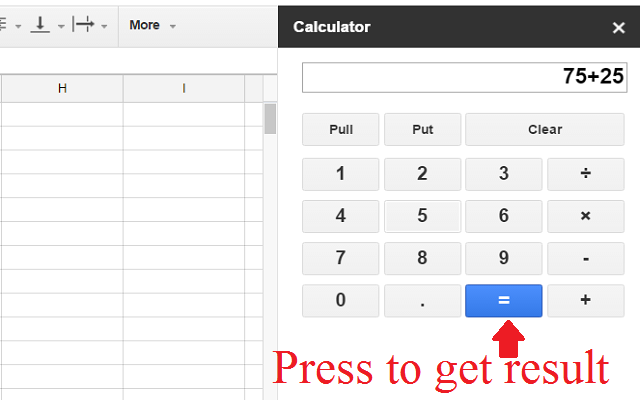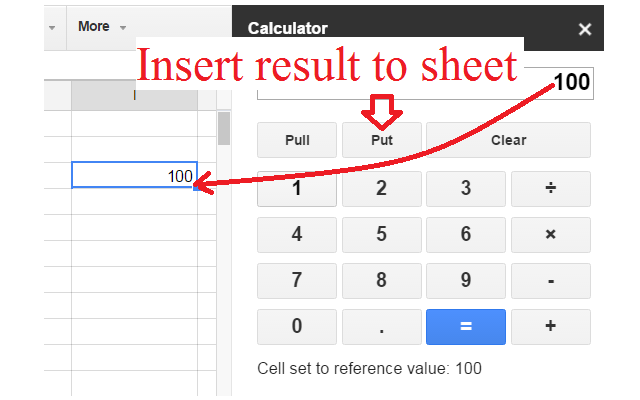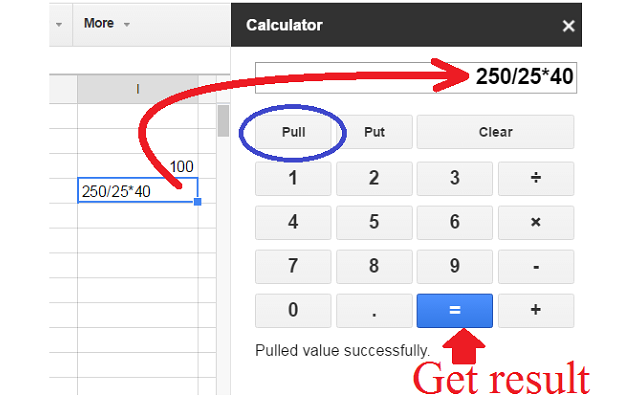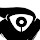Help & Support
Send FeedbackCalculator
A simple calculator and converter for spreadsheet.
5,566 users
Works with
InstallOverview
```A simple calculator for a spreadsheet. Free calculator to convert between the common length, temperature, weight units.
- Add, subtract, multiply and divide.
- Convert from Fahrenheit to Celsius, Convert from Celsius to Fahrenheit, Convert from Pounds to Kilograms, Convert from Kilograms to Pounds, Convert from Inches to cm, Convert from cm to Inches, Convert from Kilometers to Miles, Convert from Miles to Kilometers.
- Convert spreadsheet data, convert selected cells by selecting cells then click convert buttons such as lbs to kg, kg to lbs, ℃ to ℉, ℉ to ℃,  Inch to cm, cm to Inch, Mile to km, Km to Mile.```
ReviewsA User of Calculator
September 1, 2019
Useful. Just don't need the unit conversion stuff cluttering up the interface.A User of Calculator
March 12, 2019
Just what we needed!
1-2 of 2